# Sufficient statistic

for a family of probability distributions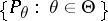or for a parameterA statistic (a vector random variable) such that for any eventthere exists a version of the conditional probability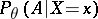which is independent of. This is equivalent to the requirement that the conditional distribution, given, of any other statisticis independent of.

The knowledge of the sufficient statisticyields exhaustive material for statistical inferences about the parameter, since no complementary statistical data can add anything to the information about the parameter contained in the distribution of. This property is mathematically expressed as one of the results of the theory of statistical decision making which says that the set of decision rules based on a sufficient statistic forms an essentially complete class. The transition from the initial family of distributions to the family of distributions of the sufficient statistic is known as reduction of the statistical problem. The meaning of the reduction is a decrease (sometimes a very significant one) in the dimension of the observation space.

In practice, a sufficient statistic is found from the following factorization theorem. Let a family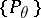be dominated by a-finite measureand letbe the density of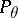with respect to the measure. A statisticis sufficient for the familyif and only if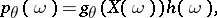(*)

where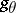andare non-negative measurable functions (is independent of). For discrete distributions the "counting" measure may be taken as, and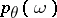in relation (*) has the meaning of the probability of the elementary event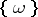.

E.g., letbe a sequence of independent random variables which assume the value one with an unknown probabilityand the value zero with probability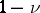(a Bernoulli scheme). Then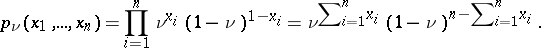Equation (*) is satisfied ifThus, the empirical frequency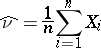is a sufficient statistic for the unknown probabilityin the Bernoulli scheme.

Letbe a sequence of independent, normally distributed variables with unknown meanand unknown variance. The joint density of the distributions ofwith respect to Lebesgue measure is given by the expression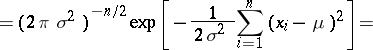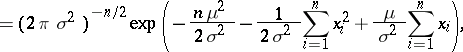which depends ononly by means of the variables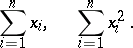For this reason the vector statisticis a sufficient statistic for the two-dimensional parameter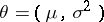. Here, the pair: sample mean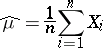and sample variancewill also be a sufficient statistic, since the variablescan be expressed in terms ofand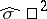.

Many sufficient statistics may exist for a given family of distributions. In particular, the totality of all observations (in the example discussed above,) is a trivial sufficient statistic. However, of main interest are statistics which permit a real reduction of the statistical problem. A sufficient statistic is known as minimal or necessary if it is a function of any other sufficient statistic. A necessary sufficient statistic realizes the utmost possible reduction of a statistical problem. In the examples discussed above the obtained sufficient statistics are also necessary.

An important application of the concept of sufficiency is the method of improvement of unbiased estimators, based on the Rao–Blackwell–Kolmogorov theorem: Ifis a sufficient statistic for the family, and if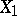is an arbitrary statistic assuming values in the vector space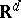, then the inequalitywhere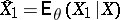is the conditional expectation of the statisticwith respect to(which is in fact independent ofby virtue of the sufficiency of), holds for any real continuous convex functionon. Often the loss functionis taken to be a positive-definite quadratic form on.

A statisticis said to be a complete statistic if it follows from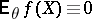,, thatalmost surely with respect to,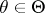. A corollary of the Rao–Blackwell–Kolmogorov theorem states that if a complete sufficient statisticexists, then it is the best unbiased estimator, uniformly in, of its expectation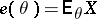. The examples above describe such a situation. Thus, the empirical frequency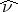is the uniformly best unbiased estimator of the probabilityin the Bernoulli scheme, while the sample meanand the varianceare the uniformly best unbiased estimators of the parametersand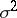of the normal distribution.

On the theoretical level it may be more convenient to deal with sufficient-algebras rather than with sufficient statistics. Ifis a family of distributions on a probability space, then a sub--algebra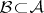is said to be sufficient forif for any eventthere exists a version of the conditional probability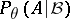which is independent of. A statisticis sufficient if and only if the sub--algebra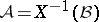generated by it is sufficient.